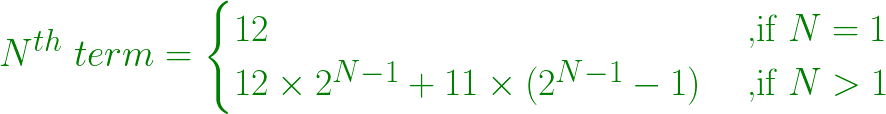# Find the Nth term in series 12, 35, 81, 173, 357, …

• Last Updated : 18 Mar, 2021

Given a number N, the task is to find the Nth term in series 12, 35, 81, 173, 357, …
Example:

```Input: N = 2
Output: 35
2nd term = (12*2) + 11
= 35

Input: N = 5
Output: 357
5th term = (12*(2^4))+11*((2^4)-1)
= 357```

Attention reader! Don’t stop learning now. Get hold of all the important DSA concepts with the DSA Self Paced Course at a student-friendly price and become industry ready.  To complete your preparation from learning a language to DS Algo and many more,  please refer Complete Interview Preparation Course.

In case you wish to attend live classes with experts, please refer DSA Live Classes for Working Professionals and Competitive Programming Live for Students.

Approach:

• Each and every number is obtained by multiplying the previous number by 2 and the addition of 11 to it.
• Since starting number is 12.

```1st term = 12
2nd term = (12 * 2) / 11 = 35
3rd term = (35 * 2) / 11 = 81
4th term = (81 * 2) / 11 = 173
And, so on....```
•
• In general, Nth number is obtained by formula:•

Below is the implementation of the above approach:

## C++

 `// C++ program to find the Nth term``// in series 12, 35, 81, 173, 357, ...` `#include ``using` `namespace` `std;` `// Function to find Nth term``int` `nthTerm(``int` `N)``{``    ``int` `nth = 0, first_term = 12;` `    ``// Nth term``    ``nth = (first_term * (``pow``(2, N - 1)))``          ``+ 11 * ((``pow``(2, N - 1)) - 1);` `    ``return` `nth;``}` `// Driver Method``int` `main()``{``    ``int` `N = 5;``    ``cout << nthTerm(N) << endl;` `    ``return` `0;``}`

## Java

 `// Java program to find the Nth term``// in series 12, 35, 81, 173, 357, ...``class` `GFG``{` `// Function to find Nth term``static` `int` `nthTerm(``int` `N)``{``    ``int` `nth = ``0``, first_term = ``12``;` `    ``// Nth term``    ``nth = (``int``) ((first_term * (Math.pow(``2``, N - ``1``)))``        ``+ ``11` `* ((Math.pow(``2``, N - ``1``)) - ``1``));` `    ``return` `nth;``}` `// Driver code``public` `static` `void` `main(String[] args)``{``    ``int` `N = ``5``;``    ``System.out.print(nthTerm(N) +``"\n"``);``}``}` `// This code is contributed by Rajput-Ji`

## Python3

 `# Python3 program to find the Nth term``# in series 12, 35, 81, 173, 357, ...` `# Function to find Nth term``def` `nthTerm(N) :` `    ``nth ``=` `0``; first_term ``=` `12``;` `    ``# Nth term``    ``nth ``=` `(first_term ``*` `(``pow``(``2``, N ``-` `1``))) ``+` `\``            ``11` `*` `((``pow``(``2``, N ``-` `1``)) ``-` `1``);` `    ``return` `nth;` `# Driver Method``if` `__name__ ``=``=` `"__main__"` `:` `    ``N ``=` `5``;``    ``print``(nthTerm(N)) ;` `# This code is contributed by AnkitRai01`

## C#

 `// C# program to find the Nth term``// in series 12, 35, 81, 173, 357, ...``using` `System;` `class` `GFG``{` `// Function to find Nth term``static` `int` `nthTerm(``int` `N)``{``    ``int` `nth = 0, first_term = 12;` `    ``// Nth term``    ``nth = (``int``) ((first_term * (Math.Pow(2, N - 1)))``        ``+ 11 * ((Math.Pow(2, N - 1)) - 1));` `    ``return` `nth;``}` `// Driver code``public` `static` `void` `Main(String[] args)``{``    ``int` `N = 5;``    ``Console.Write(nthTerm(N) +``"\n"``);``}``}` `// This code is contributed by PrinciRaj1992`

## Javascript

 ``
Output:
`357`

My Personal Notes arrow_drop_up